You can give weekly feedback to your teacher about how you are getting on with your home learning by submitting the form called Home Learning Contact form which you can find by clicking on the tile on your j2e launch page. Don't forget to look at J2Stars to see if you have been left any points.

Friday 10th July 2020

Good morning. Today is the final day for GCSE home learning. Those of you, who have persevered with the GCSE curriculum independently at home have worked extremely hard to solve some complex problems. I am sure this experience will help you to excel in maths at secondary school.

This week you have been learning how to calculate using the standard index form. Today I would like you to apply what you have learnt throughout the week by clicking on the following link and playing the matching up game. You will need to work out your answers in your home learning book before matching the correct answers. Good luck!

Thursday 9th July 2020

Good morning!

Yesterday you practiced adding and subtracting using standard index form. Today you are going to learn how to multiply and divide using the standard index form.
Watch the following video carefully.

Activity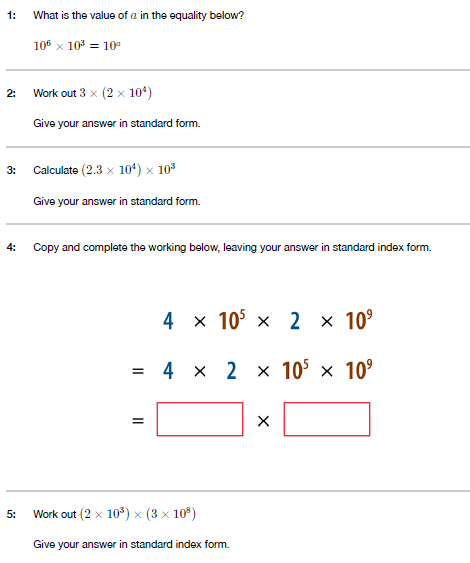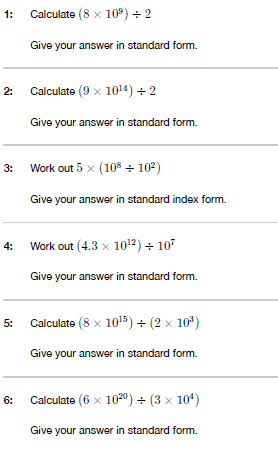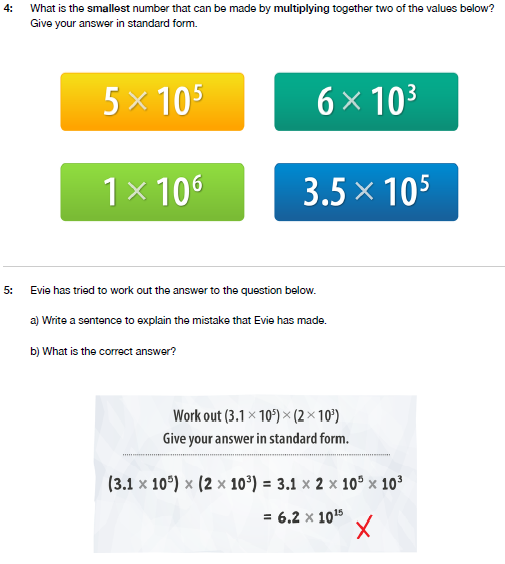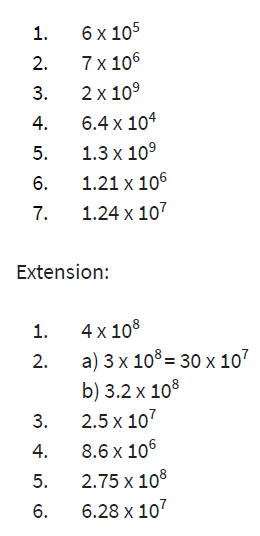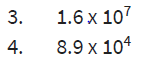Wednesday 8th July 2020

Good morning! Well done to those children who have completed the mymaths activities from the previous day. I have awarded stars for both mymaths activities.

Today you are going to practice calculating with standard index form.
Watch the following video where you will learn how to add and subtract using standard index form.

Activity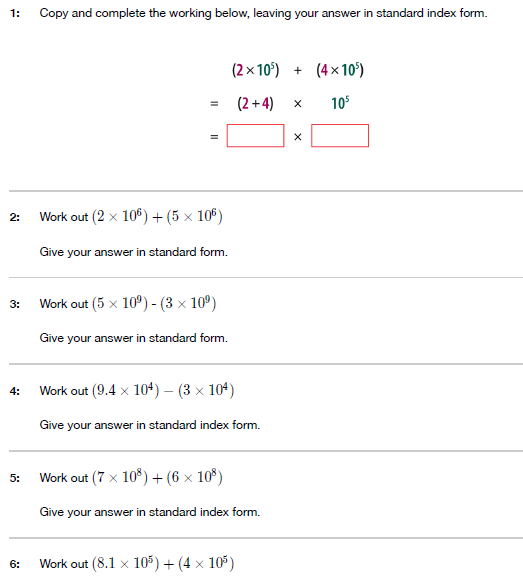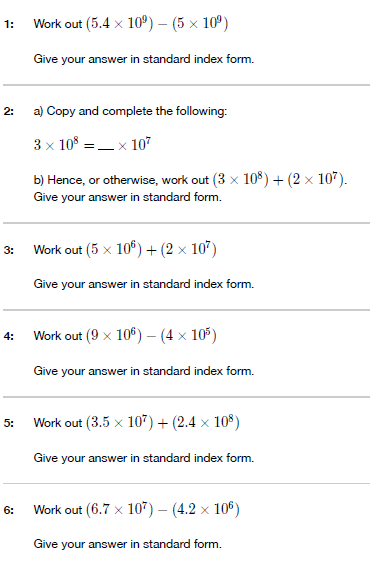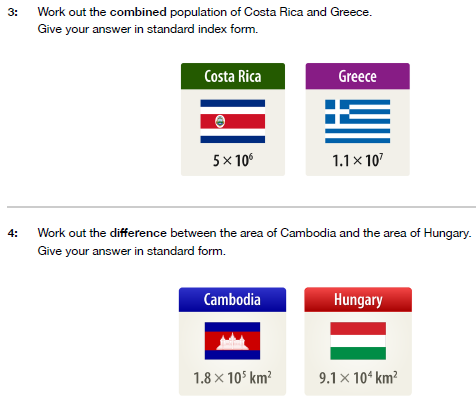Tuesday 7th July 2020

Good morning!

Yesterday we looked at how to write large and small numbers in standard index form.
Today you are going to apply what you have learnt to answer the questions on mymaths. Read through the following lessons carefully and answer the questions as accurately as you can. If you get below 80%, have another try to improve your scores.

Writing large numbers in standard index form

Writing small numbers in standard index form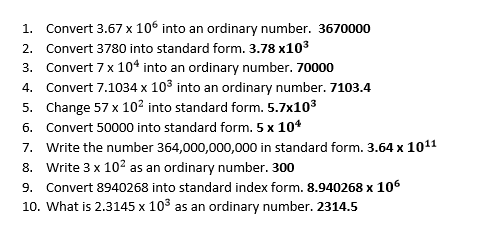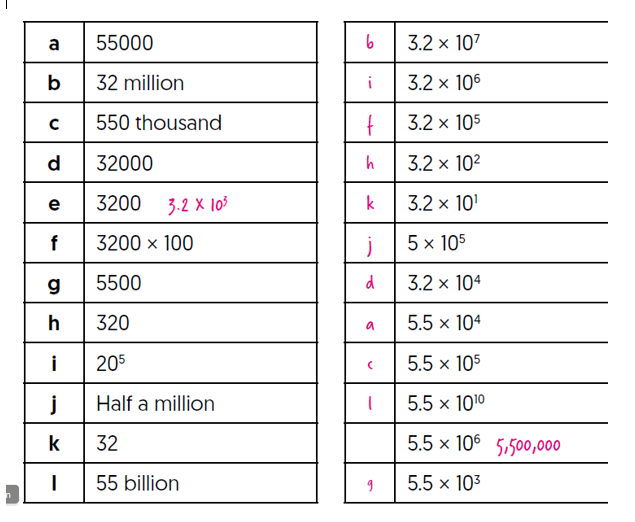Monday 6th July 2020

Good morning! I hope you have enjoyed your weekend.

This week you will be learning how to write numbers in standard index form.
Today you will be learning how to write small and large numbers using the standard form.

Click on the following link and scroll down to find week 7 w/c 8th June. Watch lesson 3 and lesson 4 where you will recap on how to write large and small numbers in standard index form. Watch the videos twice if you need to. Remember to scroll down to find week 7 w/c 8th June first!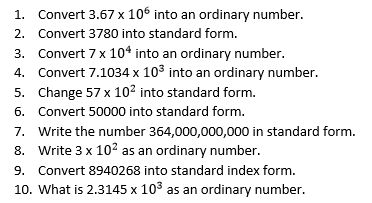Now play this match up game. Match up each integer with the equivalent value. One has been done for you. Two of the values don’t match up!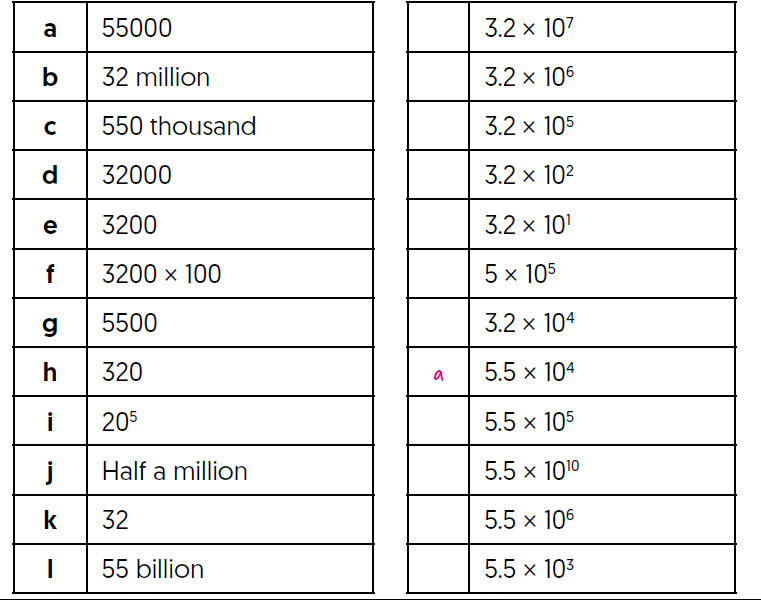Friday 3rd July 2020

Good morning! Today you are going to answer reasoning questions involving calculations with roots and indices.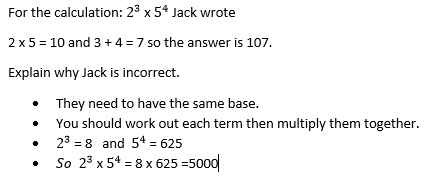Thursday 2nd July 2020

Good Morning!

Today you are going to apply what you have learnt and answer the questions on page 351 exercise 17.1S of your GCSE text book. Read through the information on page 350 first.

I have also set the mymaths work on indices.

Wednesday 1st July 2020

Good morning! Now that you have worked through the lessons on how to multiply and divide powers with the same base, you are going to apply your skills by answering the questions on mymaths.
Click on the mymaths link and read the information carefully to answer questions based on how to calculate with indices and roots.

Tuesday 30th June 2020

Good morning!

Yesterday you practiced how to multiply powers using the same base. Today you are going to practice how to divide powers using the same base.

Click on the following link. This will take you to the BBC home learning page where you can read the information and watch the videos, which will show how to divide powers using the same base. Remember to click on activity 1, where you can answer the questions.

Monday 29th June 2020

Good morning! I hope you have been enjoying the weather this week.

Today you are going to revise how to calculate using roots and indices.

Click on the following link. This will take you to the BBC home learning page where you can read the information and watch the videos, which will show you how to multiply powers using the same base. Remember to click on activity 1, where you can answer the questions.

Friday 26th June 2020

Good morning. Well done to those of you, who have been completing the activities on mymaths. I am very impressed with your scores.

Today you are going to solve problems involving direct proportion.

Read the example page on 454 of your GCSE book and answer the questions on page 455 exercise 22.2A.
Once you have finished, turn to the back of your book to find the answers and mark your work.

Thursday 25th June 2020

Good morning. Yesterday you completed a lesson on mymaths that taught you how to work out direct proportion.

Read through the following power point to help you re-cap on how to work out direct proportions.

Once you have finished, turn to the back of your book to find the answers and mark your work.

Wednesday 24th June 2020

Good morning.

Take the following quiz to recap on unitary methods.

Today you are going to move onto working out the direct proportion.
Read through the lesson on direct proportion carefully and answer the questions. Attempt the lesson more than once if you have a score below 80%.

Tuesday 23rd June 2020

Good morning. Yesterday you worked through the lesson on mymaths, which taught you how to use the unitary method. Today you are going to answer questions based on what you have learnt.

Here is an example:

A teacher pays £27.60 for 6 calculators. How much does he pay for 15 calculators at the same price each?
• 6 calculators cost £27.60
• To find the cost of 1 calculator, you divide £27.60 by 6 using your calculator =£4.60
• So £4.60 is the cost of 1 calculator. To find the cost of 15, you multiply £4.60 x 15 =£69

1. Abby travelled for three hours on the motorway and covered 190 miles.
a) How far would Abby travel in 5 hours at the same average speed?
b) How far would she travel in half an hour?

2. Dave drove 246 miles and used 25.4 litres of diesel.
a) How many litres of diesel would he need for a 400 mile journey?
b) How far can he go on 10 litres of diesel?

3. Here are some prices for Minty toothpaste

 Size Amount of toothpaste Price Small 50 ml 99p Standard 75 ml £1.10 Large 100 ml £1.28

Which sizes gives the most toothpaste for one penny? Show your working out.

4. These are the prices for different packs of bird seed.

 Pack size Price 5.50kg £15.65 12.75kg £28.00 25.50kg £53.00

a) Find the cost for 1kg of bird seed for each of the different packs.
b) Which pack offers the best value for money?
c) Find the cost of a 25.50kg pack if the price per kilogram was the same as for the 5.50kg pack.

5. The weights of objects on other planets are proportional to their weights on Earth. A person weighing 540 newtons on Earth would weigh 90 newtons on the moon and 1350 newtons on Jupiter.a) What would a teenager weighing 360 newtons on Earth weigh on Jupiter?
b) What would a rock weighing 10 newtons on the moon weigh on Earth?
c) What would an astronaut weighing 130 newtons on the moon weigh on Jupiter?

6. Sajid worked for 8 hours and was paid £30.
a) How much will he be paid for working 10 hours at the same rate of pay?
b) Copy and complete this table. Plot the values in the table as points on a graph, using the numbers of hours worked as the x co-ordinates and the money earned as y co-ordinates.
c) Show how to use the graph to find out how much Sajid earns in 5 hours.

The answers will be given tomorrow for you to correct yourself.

Monday 22nd June 2020

Good morning. I hope you have enjoyed your weekend.

Today we are going to move onto the Ratio and Proportion topic.

Read through the lesson on using the unitary method carefully and answer the questions. Attempt the lesson more than once if you have a score below 80%.

Friday 19th June 2020

Good morning. Well done for completing the Mymaths activity from yesterday. I have awarded stars for this.

Today is our final day of calculating compound measures. This has been a tricky topic so well done to those of you, who have persevered. You will learn more about this in Secondary school.

Today you are going to revise what you have learnt this week.

Thursday 18th June 2020

Good morning. Well done for completing the Mymaths activity from yesterday. I have awarded stars for this.

Today you are going to recap on how to work out the density. Use this formula triangle to help you work out the density, mass and volume.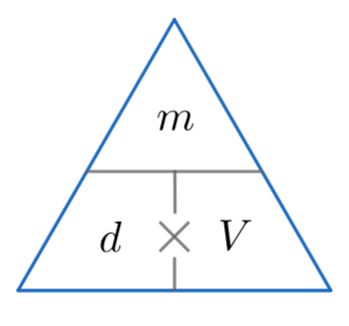Remember, to work out the volume, you multiply the length, width and height.

Wednesday 17th June 2020

Good morning.
Today you are going to recap on how to work out the speed. Use this formula triangle to help you calculate speed, time and distance.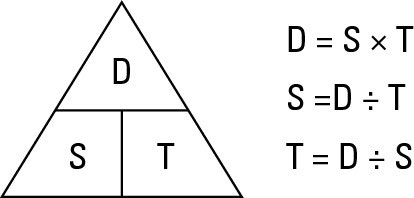Tuesday 16th June 2020

Good morning. Well done to those children, who uploaded their work onto J2e. You have received stars for this.

Today you are going to use the formulas that you have learnt for calculating speed, density and pressure to answer the questions from your GCSE book.
Turn to page 448 to read through the examples carefully and answer the questions on page 449 exercise 22.1S.

You may want to watch the three videos again to recap on how to work out speed, density and pressure.

Remember to use a calculator. Once you have finished, turn to the back of your book to mark your answers.

Monday 15th June 2020

Good morning, I hope you have had a good weekend.

Today we are going to learn how to calculate compound units. You are going to learn how to work out the speed, density and pressure

.
Speed = How fast or slow something travels.
Density = Density is a word we use to describe how much space an object or substance takes up (its volume) in relation to the amount of matter in that object or substance (its mass).
Pressure = The exertion of force upon a surface by an object.

Watch the following videos and attempt the questions that accompany each video. There are 3 different videos that explain how to measure speed, density and pressure. Remember to scroll down and watch all three.

Activity 1
In your books, draw formula triangles for speed, density and pressure. You can design a poster instead! Write down how to calculate each part of the triangle. Here is an example of a formula triangle for speed. The D stands for distance, the T stands for time and the S stands for speed.Now draw a formula triangle for density and pressure.

Activity 2
Read through the following information and answer the questions at the bottom of page 70. Remember to use a calculator.

You can upload pictures of activity 1 and 2 on j2e. You ca get extra stars for interesting presentation and correct answers.

Friday 12th June 2020

Good Morning.

Today is the final day of our probability topic. Click on the following Mymaths link to answer the revision questions for the topic.

Thursday 11th June 2020

Good morning Year 6. I have now set the mymaths from yesterday so please log on to complete. I have given you a week to complete this so you can do this anytime before next week.

Today you are going to learn what mutually exclusive events are. Click on the following link and watch the video that offers an explanation of mutually exclusive events.

Remember the rule, in any given probability situation, the total probability always equals 1.

Activity
Read through the examples on page 174 of your GCSE book and answer the questions on page 175 exercise 8.4S. When you have finished, mark your work using the answers at the back of your book.

Wednesday 10th June 2020

Good Morning. Well done to those children who uploaded their probability work on J2e on Monday. I have awarded stars for this.

Carefully watch the lesson on Mymaths and log in to complete the activities. The first part recaps on how to calculate relative frequencies and the second part of the lesson introduces how to work out expected outcomes.

An expected outcome is the number of times you expect an event to occur. To work out the expected outcome you multiply the probability by the number of trials. Probability of an event X number of trials.

Relative frequencies are an estimate of an event occurring based on previous experiments. Remember, to work out the relative frequency, you divide the number of times the event occurs by the number of trials altogether. For example, I throw a piece of toast in the air 40 times and it lands butter side up 24 times. Calculate the relative frequency. You use your calculator to divide 24 by 40 and the answer is 0.6 or 60%.

Tuesday 9th June 2020

Good morning.

Yesterday, you learnt how to calculate expected outcomes. Watch the following video again to remind yourself how to calculate this.

Activity
Turn to page 166 of your GCSE book and read through the examples. Now answer the questions on page 167 exercise 8.2S. When you have finished, mark your work using the answers at the back of your book.

Monday 8th June 2020

Good Morning! I hope you have had a good weekend.

Today you are going to work out expected outcomes of different events. If you know the probability of an event, you can calculate how many times you expect an outcome to happen.

Expected outcome = Number of trials X probability of an event.

Estimate the number of times you expect 2 to occur if you roll the dice 30 times.
The probability of scoring a 2 on a dice is 1/6 as there is one two out of 6 numbers.
To calculate the expected outcome, multiply the probability by the number of trials i.e.
1/6 x 30 = You will need to use your calculator to divide 1 by 6 first:
1 ÷ 6 x 30 = 5   On average there will be 5 of each of the numbers 1,2,3,4,5 and 6 in the 30 trials so you would approximately expect the number 2 to occur 5 times in 30 trials however this may not exactly happen when you actually do the experiment.

If you get a decimal number in your answer, simply round this to the nearest whole number.

When conducting probability experiments, it is important to do the experiment lots of times to get a result close to the theory (what you think might happen).

Click on the link and watch the video that explains different ways of working out the expected outcome.

Expected outcomes experiment.

Calculate the expected number of heads if you spin a fair coin. Expected outcome = Number of trials X probability of an event.

a) 8 times
b) 80 times
c) Calculate the expected outcome of landing on heads when you spin a coin 60 times.
d) Now conduct the experiment yourself and record your results in a table. Were your actual results close to your expected outcome prediction?
e) Do the same experiment more than once. See if you start to get similar numbers close to your expected outcome.

Friday 5th June 2020

Good Morning. Well done for completing Mymaths yesterday, I have awarded stars on J2 stars.

Yesterday you completed the lesson on how to calculate Relative Frequencies on Mymaths.

Let's recap on how to work out the relative frequency. A spinner is spun 200 times. Red appears 25 times. What is the relative frequency of red occurring?

To work out the relative frequency, you divide the number of times red appears in the first experiment by the number of spins altogether. The red came up 25 times so you would divide 25 by the total number of spins, which is 200. The answer is 0.125 or 12.5%

Today I would like you to turn to page 162 of your GCSE maths book and read through the information and the examples on how to calculate the relative frequencies again before you answer the questions on page 163 Exercise 8.1S. Once you have completed the questions, turn to the back of your book to find the answers and mark your work.

Remember, relative frequencies can be represented at a decimal, fraction and a percentage.

Thursday 4th June 2020

Good Morning.

Today you are going to learn how to calculate Relative Frequencies.
Carefully watch the lesson on Mymaths and log in to complete the activities.

1a) HA   HO   HP   BA   BO  BP  CA  CO  CP  EA  EO  EP
b)

 Ham Beef Cheese Egg Apple HA BA CA EA Orange HO BO CO EO Pear HP BP CP EP

C) i)   1/12  ii) 3/12 or 1/4

2a)

 1 2 3 4 5 6 1 2 3 4 5 6 7 2 3 4 5 6 7 8 3 4 5 6 7 8 9 4 5 6 7 8 9 10 5 6 7 8 9 10 11 6 7 8 9 10 11 12

b) i) 1/36    ii) 3/36 or 1/12     iii) 6/36 or 1/6    iv) 18/36 or  ( 1)/2

3a)

 1 2 3 4 5 6 1 1 2 3 4 5 6 2 2 4 6 8 10 12 3 3 6 9 12 15 18 4 4 8 12 16 20 24 5 5 10 15 20 25 30 6 6 12 18 24 30 36

b)i) 1/36    ii) 4/36 or 1/9  iii) 17/36  iv) 9/36 or 1/4

Wednesday 3rd June 2020

Good Morning. Well done for completing Mymaths yesterday, I have awarded stars on J2 stars.

Here are the answers to yesterday’s questions.

Tracey buys a drink and an ice cream from a shop.
The drinks available are orange juice (O), lemonade (L) and water (W).
The ice creams available are vanilla (V), strawberry (S) and chocolate (C).

a) Make a list of all the possible combinations of drink and ice cream.
Answer: Try to be systematic (this means an organised method) and list the options by letter:

OV   OS   OC   LV   LS   LC   WV   WS   WC

b) Show the same information on a two-way table.

 Vanilla Strawberry Chocolate Orange Juice OV OS OC Lemonade LV LS LC Water WV WS WC

c) Find the probability of Tracey buying:

i) Lemonade and strawberry ice cream.

Answer: The table shows there are nine possible combinations of drink and ice cream.  Lemonade and strawberry ice cream is one of these nine. So the probability = 1/9

ii) Orange juice and an ice cream other than vanilla.
Answer: The options are OS or OC. This is two out of nine so the probability = 2/9

Activity

1) Trevor is having lunch at his office restaurant. He chooses a sandwich and a piece of fruit.
The sandwich choice is: ham (H), beef (B), cheese (C) or egg (E).
The fruit choice is: Apple (A), orange (O) or pear (P).

a)Make a list of all the possible combinations of sandwich and fruit he could choose.
b)Show this information in a two-way table.
c)What is the probability that Trevor chooses:
i)Ham sandwich and an apple?
ii)An egg sandwich?

2)Two ordinary dice are rolled. The scores on each side are added together.

a) Create a two-way table to show all the possible results. Remember to be systematic when organising your results.
b) Use your table to find the probability of scoring:

i)2
ii)4
iii)More than 9
iv)Even number

3)Two ordinary dice are rolled. This time the scores on each side are multiplied together.

a)Create a two-way table to show all the possible results. Remember to be systematic when organising your results.
b)Use your table to find the probability of scoring:

i)1
ii)12
iii)Less than 10
iv)Odd number

Tuesday 2nd June 2020

Good Morning. Well done for completing Mymaths yesterday, I have awarded stars on J2 stars.

Today you are going to work out all the different possibilities when two or more events occur at the same time. You can do this by either creating a list or a two-way table.
Carefully watch the lesson on Mymaths and log in to complete the activities.

Now that you understand how to work out possible outcomes using a list or a two-way table, try these questions and answer them in your maths home learning book. The answers will be posted tomorrow.

1. Tracey buys a drink and an ice cream from a shop.
The drinks available are orange juice (O), lemonade (L) and water (W).
The ice creams available are vanilla (V), strawberry (S) and chocolate (C).

a) Make a list of all the possible combinations of drink and ice cream.
b) Show the same information on a two-way table.
c) Find the probability of Tracey buying:
i) Lemonade and strawberry ice cream.
ii) Orange juice and an ice cream other than vanilla.

Monday 1st June 2020

Good Morning! This is Mrs Patel. I hope you have all had a lovely half term and a well-deserved break.

This week, we are going to re-visit the topic on probability as this was an area that was particularly tricky when we first came across it.

The vocabulary for the topic is: Chance, possible, impossible, likely, unlikely, relative frequency, expected outcomes, theoretical probability, mutual and exclusive events.

Today, you are going to start off by understanding what basic probability is.
Carefully watch the lesson on Mymaths and log in to complete the activities.

Friday 22nd May 2020

Good morning everybody.

Today you will be using your white GCSE workbook.

Please turn to pages 32 and 33: sequences.

Complete Q1 to Q10.

Make sure you read the useful tips in the bubbles next to some of the questions. They are very helpful.

For example, with Q3, write the difference between each of the terms above the numbers. This will help you to spot how the difference between each of the terms is changing.

When you get to Q9, writing the terms in a function machine can be helpful in finding the nth term.

For example:

 In (n) Out f(n) 1 2 2 4 3 6 4 8

Here, we can clearly see that the second number is double the first in all cases.

So the expression is 2n.

Have a restful half term break everybody. I'm looking forward to seeing your holiday projects after the holiday!

Thursday 21st May 2020

So far this week we have learnt:

• To generate sequences using a term to term and a position to term rule.
• To describe a sequence using a term to term rule.

For example:

Sequence: 3, 8, 13, 18, 23,

Term to term rule: add 5

Position to term = T(n) = 5n - 2

Today we are going to:

• Generate a sequence applying the term to term rule to the previous term.
• Find a particular term by substituting n (the term's position) into the nth term formula, T(n).

Look at the worked examples on P432 21.1 in your GCSE textbook.

Now complete Exercise 21.1A Q 1to 5 in your home learning book.

Use the answers at the back of the book to check your work when you have finished.

Remember to send your teacher an email if you need to. They will pass it onto me.

Well done everybody!

Wednesday 20th May 2020

Good morning everybody.

Open your GCSE textbooks to Page 430, 21.1.

Sequences can be generated and described using term to term rules, for example 20,13, 6,-1,-8.

The term to term rule here is subtract 7.

Sequences can also be generated and described using position to term rules.

With position to term rules you will be given a rule for a sequence.

For example: T(n) = 2n + 3

T(n) is the nth term of the sequence.

n is the position of the term in the sequence.

Think of this as just like our function machines in school.

2n + 3

 in out 1 5 2 7 3 9 4 11 5 13

T(1) = 2 x 1 + 3 = 5           T(1) is the first term in the sequence

T(2) = 2 x 2 + 3 = 7           T(2) is the second term in the sequence and so on.

Now complete exercise 21.1S Q 1 to 5 in your home learning book.

When you have finished, check your answers using the back of the book.

Tuesday 19th May 2020

Well done for your hard work on MyMaths yesterday. I have awarded stars on J2 stars.

Today we are going to continue the work on algebra and sequences before we move onto some practice using the GCSE textbook.

Watch the MyMaths lesson below carefully.

Then login to MyMaths and complete the online homework.

Monday 18th May 2020

Good morning everybody.

Today we are going to start a new topic on sequences.

Key vocabulary: sequence, term, position.

Carefully watch the lesson below on MyMaths.

Then login to MyMaths and complete the online homework.

Friday 15th May 2020

Good morning.

Today you will be using your GCSE Maths workbook.

You will be revising a range of charts and graphs.

Open your workbook to p110 and complete Q 1 to 9.

Tips:

Q3: Remember that each bar represents 100%. Use the key to make sure that you are looking at the correct marital status and correct gender.

The first graph shows males, the second females.

Q6: Start with the easy bits first, ie where there is only one piece of missing information in the row or column. The total column looks a good place to start!

Q7: Revise the meaning of mode/modal, median and mean before you start. (GCSE textbook P84).

Q8b: To descibe the trend you need to ignore the seasonal pattern. The seasonal pattern is that people buy more suncream in the spring and summer months. Plot the points, join with a ruler and look at the trend, how the data changes over time.

I will share the answers on Monday.

Have a lovely weekend everyone!

Thursday 14th May 2020

Good morning everybody. Well done for your hard work on MyMaths yesterday.

Open your GCSE textbook to P342 and look at 16.4.

Look carefully at the how to section.

Today you will be:

1. Drawing axes and using a suitable scale to label the axes.
2. Plotting the data from a given table as coordinate pairs. Joining the plotted points with straight lines.
3. Discussing short term and longer term trends and seasonal variations.

Remember that the trend is how the data changes over time.

Read carefully the worked example on P342.

Look at the vertical axis. Remember that it does not have to start from 0.

The time series graph clearly shows that Jenny's gas bills are highest in the winter months, January to March and October to December.

If you look at the cost of the bills and compare 2003 to 2004, you can see that there is a slight trend for the bills to rise from year to year.

Now look at Ex 16.4A.

Complete Q 1,2 and 3 in your home learning book. Remember to comment on any patterns in the data for each graph.

You can check your answers at the back of the book when you have finished.

Wednesday 13th May 2020

Good morning everybody.

Today we are going to learn how to interpret time series data in charts and graphs.

We will also start to understand trend lines which can give us information about the overall pattern or behaviour of the data.

A new key word for today is anomaly. This is where a data value does not fit in with the rest of the data.

Watch the lesson carefully on MyMaths.

Then login and complete the online home learning.

Tuesday 12th May 2020

Open your GCSE textbook to P340, 16.4.

Key information:

You can use a line graph to show how data changes as time passes.

The data can be discrete or continuous.

Discrete data can only take exact values and is usually collected by counting.

Continuous data can take any value and is collected by measuring.

Time is always the horizontal axis. Time could be seconds, minutes, hours, days, weeks, months or years.

Look carefully at the time series graph at the top of the page. The temperature has been measured every minute and the temperature plotted on the graph.

Now have a look at the worked example on P340.

Look carefully at the vertical scale. Can you see a wobbly line between 0 and 5? This is a break in the axis and shows us that the scale goes straight from 0 to 5. It gives more space to values on the y axis and shows patterns and trends more clearly.

Open your home learning books and complete Exercise 16.4S, Q 1,2,3 and 4.

Don't forget to label your axes.

Monday 11th May 2020

Good morning everybody.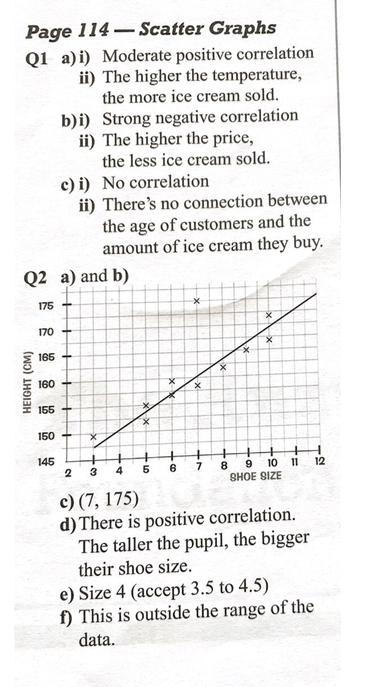Today we are going to look at line graphs. By the end of this week I would like you to be able to interpret and construct line graphs for time series data.

Key vocabulary: data, line graph, time series graph, axes, trends.

Watch the following lesson carefully on MyMaths.

Then complete the online homework.

Thursday 7th May 2020

Good morning everybody.

Thank you to the pupil who emailed Mrs Hunt Stewart to say that the recent work on inequaliities was challenging. Don't worry! Inequalities can be very tricky. We will return to this topic and practice more soon.

Today is our last day on scatter graphs.

Open your GCSE mathematics workbook to P114.

Complete the two questions.

I will share the answers on Monday.

Have a lovely bank holiday weekend.

Wednesday 6th May 2020

Open your GCSE text book to P336, 16.3.

Look carefully at the three scatter graphs showing positive, negative and no correlation.

Study each one and describe it.

For example, in the first one we can see that as a person gets taller, their mass increases.

Repeat for the other two and then check your thinking by reading the sentence underneath.

Read the worked example on P336 carefully.

Key learning today:

1. Points that lie outside the line of best fit and which do not fit the general pattern are called outliers.

For example, an extremely old, very rare car could be very valuable. This then may not fit the general trend of a car's age increasing and its price decreasing.

2. If two variables are corelated, it does not always mean that one causes the other.

Now turn to Exercise 16.3 on P337 and complete the questions in your home learning book.

Check your work when you have finished by referring to the answers at the back of the book.

I have not received any messages from the teachers from you this week. I assume therefore that scatter graphs are going well. Don't forget you can let your teacher know if you have found something difficult and they will let me know.

Tuesday 5th May 2020

Yesterday we learnt that a scatter graph can be used to compare two sets of data.

The data is collected in pairs and plotted as coordinates.

If the points lie roughly in a straight line, there is a correlation between the two variables.

Correlation can be:

Positive: both variables increase.
Negative: one variable increases as the other decreases.

There can also be no correlation because there is no relationship between the two variables. The points do not lie in a line but are scattered randomly over the graph.

If the points lie close to the line of best fit there is a strong correlation.

Open your GCSE textbooks to P336, Exercise 16.3.

Then complete  Exercise 16.3S on P337 in your home learning books. You can refer to the answers when you have finished to assess your work.

Monday 4th May 2020

Friday’s work on finding the mean, median and mode from a frequency table.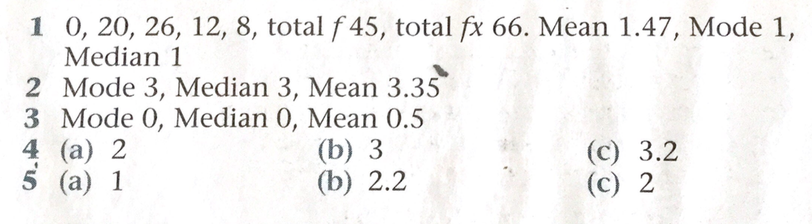Today we are going to learn about scatter graphs and correlation.

Key vocabulary: scatter graph, data, variable, correlation, positive, negative, line of best fit.

Watch the following lesson on MyMaths.

When you get to the last slide, slide 7. You will need to draw a scatter graph in your home learning book.

Make sure that you choose an appropriate scale. Think carefully about what number you will start off with when you are adding the height scale. The shortest height is 128cm. So, don’t start at 0 as there will be a lot of empty, unneeded space on your graph.

Have a go at drawing your own graph before you click the next button.

Compare your scatter graph with the one on MyMaths.

Compare the scales. Was yours appropriate?

Did you draw the line of best fit in the correct place? Did it pass through or is as close to as many of the plotted points as possible?

Now complete the online homework.

Friday 1st May 2020

Today we are going to practice calculating the mode, median and mean from a frequency table.

I have seen from your MyMaths work that some of you are finding calculating the mean a little tricky. Mrs Din also passed me a message from one of you about this.

So, look at the example below really carefully. It will guide you with your independent work.

Some shoppers were asked how many National Lottery tickets they bought last week. The results are shown in this table.

 Number of tickets x Number of shoppers. frequency (f) 0 2 1 7 2 5 3 2 4 0 5 3 6 1

Have a look at this for a minute and make some sense of it.

2 shoppers who were asked the question did not buy any tickets.

5 shoppers who were asked the question bought 2 tickets last week.

1. Which number of tickets is the mode?

The mode is the value that occurs most often. In a frequency table this is the item of data which has the highest frequency. The highest frequency is 7 so for this set of data the mode is 1. More people bought 1 ticket than any other number of tickets.

2. Work out the mean number of tickets.

The mean is the total number of tickets divided by the total number of shoppers.

So we have to add an additional column to our table to calculate the total number of tickets.

 Number of tickets x Number of shoppers frequency (f) Frequency (f) times by the number of tickets (x) f times x 0 2 0 1 7 7 2 5 10 3 2 6 4 0 0 5 3 15 6 1 6 Totals 20 44

The mean is the total number of tickets (44) divided by the total frequency (20).

So 44 divided by 20 = 2.2

3. Find the median number of tickets.

The median is the middle value of the data. There are 20 shoppers altogether.

The median value is between 10 and 11. (These two numbers are in the middle).

2 shoppers bought 0 tickets.

7 shoppers bought 1 ticket.

This is 9 shoppers altogether so far. This means that shopper 10 and shopper 11 bought 2 tickets.

The median number of tickets is 2.

Now have a go at the following 4 questions.

Follow the steps above carefully to help you calculate the mean, median and mode for each set of data.

Pass a message to your teacher for me via the email or home learning form if you need to.

Well done everybody for  all your hard work.

I will share the answers on Monday.Thursday 30th April 2020

Good morning everybody.

Today we are going to learn how to find the mode and the median from data organised in a frequency table.

The mode is the value that occurs the most.

The median is the value in the middle when the values are arranged in order of size.

So, for the following set of data values, think about which is the mode and the median.

2,3,4,4,4,6,7,8,12.

4 is the mode as it occurs the most.

4 is also the median. Look at the bold 4. There are 4 values either side of it.

1,1,2,2,3,5,7,8,10,11.

There are 2 modes: 1 and 2 as these values occur the most.

There are 10 values. 1,1,2,2,3,5,7,8,10,11.

3 and 5 are in the middle so we have to find the number halfway beween them.

4 is the median value.

Watch the following lesson carefully on MyMaths.

Wednesday 29th April 2020

Good morning everybody!

Today we are going to learn how to calculate the mean from a frequency table.

The frequency tables today do not have grouped data.

However, you need to know how to find the mean when a set of data shows the number of times a value occurs. For example:

In a survey, the number of eggs in seagulls' nests in June was counted. The table below shows the results.

 Number of eggs x Frequency f 0 17 1 12 2 23 3 37 4 18 Total 107

So we can see that 0 eggs were found on 17 occasions,1 egg was found on 12 occasions, 2 eggs were found in seagulls' nests on 23 occasions and so on.

How do we find the mean?

 Number of eggs x Frequency  f Frequency x number of eggs f times x 0 17 0 1 12 12 2 23 46 3 37 111 4 18 72 Totals 107 241

The mean is the total number of eggs (241) divided by the total number of nests (the total frequency) 107.

So, 241 divided by 107 = 2.25 eggs

Watch the lesson on MyMaths carefully.

Then complete the online homework.

Tuesday 28th April 2020

Today we are going to move onto interpreting frequency diagrams.

To interpret a frequency diagram you need to:

1. Read information from the diagram, making sure that you read the scale carefully.
2. Use the information from the diagram to answer the question.

Open your GCSE textbook to P330.

Read the recap section carefully at the top of the page.

Remember:

When there are lots of values, you can group the data into class intervals.

Discrete data can only take exact values and is usually collected by counting. E.g the number of children in 4R.

Continuous data can take any value and is collected by measuring. E.g. the height of tomato plants.

• You can use a bar chart to display grouped discrete data.

• You can use a grouped bar chart to display continuous data.

Look at the worked example carefully underneath.

Now turn to Exercise 16.1A in your GCSE book and complete questions 1 to 4 in your home learning book.

You can check the answers on p604 when you have finished.

Monday 27th April 2020

Good morning everybody.Today we are going to use our learning last week on inequalities and apply this to frequency tables.

Key vocabulary: category, group, frequency, inequality interval.

First, I would like you to watch the lesson on MyMaths below carefully.

Now open your GCSE textbook to P329 Ex 16.1S. Q 1,2 and 3.

Today you will be practising completing frequency tables. You will be grouping the data into class intervals as there are a lot of categories.

You also have to draw a bar chart or a histogram.

Look at the worked examples on P328.
A bar chart has gaps between bars. Make sure those gaps are equal width.
Remember to label axes and give your graph a title.

Look carefully at the horizontal axis on both charts.
The bar chart shows grouped discrete data. The class interval is used to describe the bar. So, 7 tomato plants grew between 1 and 5 tomatoes.

The histogram displays grouped continuous data. There are no gaps between the bars.
Look carefully at the horizontal axis and how the heights are labelled.

Keep referring to these charts as you draw your own.

Friday 24th April 2020

Good morning everybody! Well done for all your hard work this week.

Today is our last day on inequalities and I would like you to use your white GCSE AQA mathematics work book.

Turn to P34 - Inequaliities.

Remember, an empty or open circle means that the number is not included.

A closed or shaded circle means that the number is included.

I'll share the answers on Monday.

Have a lovely weekend everybody!

Thursday 23rd April 2020

Q1.                                                                          Q2.                                                                          Q3.Today we are going to work on linear inequalities.

Turn to P218 of your GCSE textbook.

Look carefully at the second example box. For each example you have to solve it and then represent the solution on a number line.

a) Here we have 3x < 15

First we have to divide both sides of the inequality by 3 to give us x.

Then we have x < 5.

Then look at the solution on the number line. An open circle has been used as 5 is not included.

b) Here we have 4x + 3 ≥ 21

First we have to subtract 3. (Remember when we solved equations using the balancing method?)
So, that gives us 4x ≥ 18

We want x on its own so we have to divide both sides by 4.
This gives us x ≥ 4.5

Then look at the solution on the number line. A closed circle is used because 4.5 is included.

c) Here we have 6 <3 (x -2) <18

(ERROR IN THE BOOK BETWEEN THE QUESTION AT THE TOP AND THE WORKED EXAMPLE)

First multiply out the brackets which will give   6 < 3x -6 < 18

Next we want to remove the - 6 so balance  by adding 6

12 < 3x  <  24   (We have added 6 to both sides).

Now we want x on it own so we have to divide by 3.

4 < x < 8

Look at the number line.  Open circles are used because 4 and 8 are not included. So x = 5, 6,and 7.

Now have a go at P219 Ex 10.5S Questions 1 to 5.
Check your work using the answers in the back of the book.

If you have any questions, pass a message via the contact form to your teacher or using the new class email. Miss Din passed me a message from Angad yesterday and I have added a task on MyMaths as requested.

Good luck everyone.

Wednesday 22nd April 2020

Today I would like you to practice inequalities.

You may be asked to show them on a numberline.Have another look at these examples to help you to remember what to do.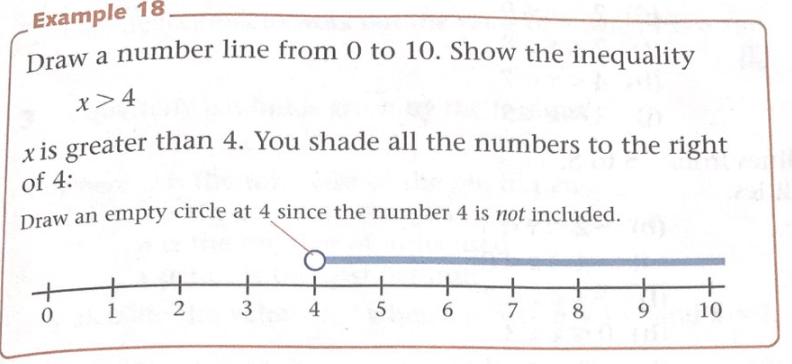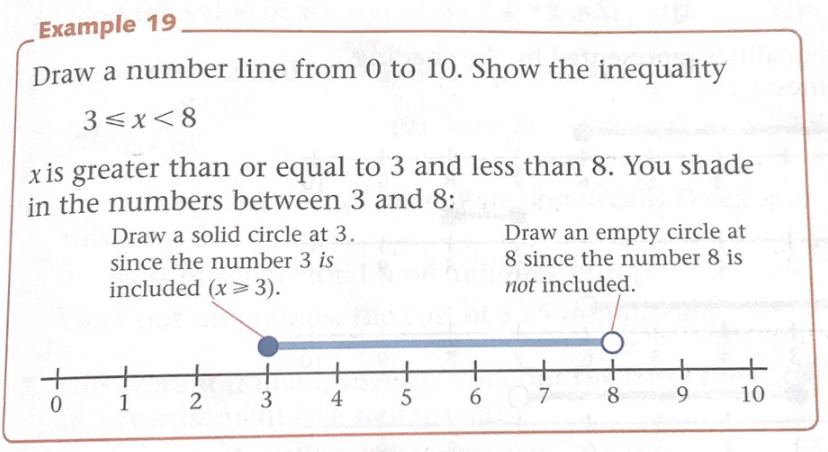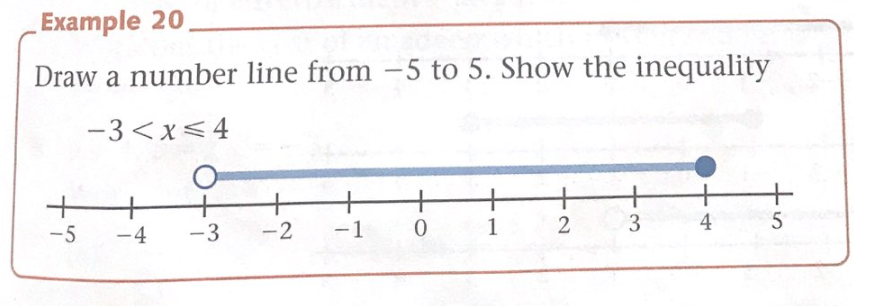Remember, an empty circle shows that the number is NOT included.

A solid circle shows that the number IS included.

Now have a go at these questions in your home learning book. I will show you the answers tomorrow.

Question 1.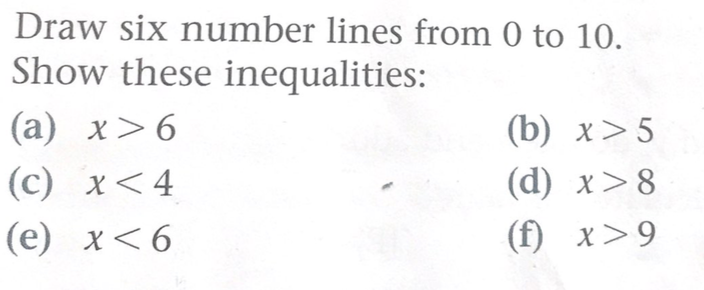Question 2.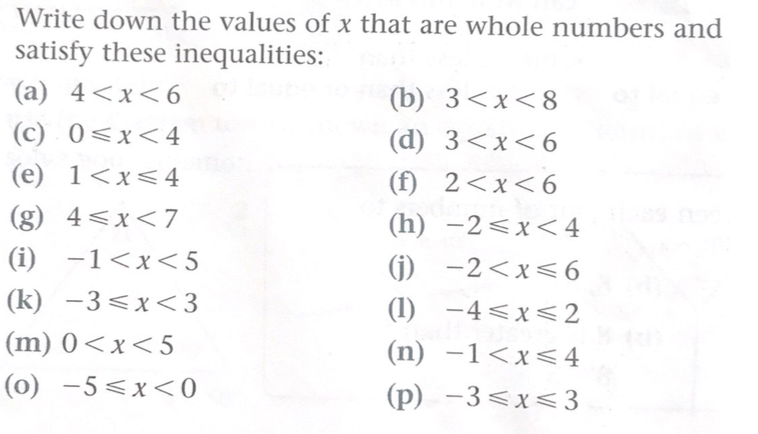Question 3.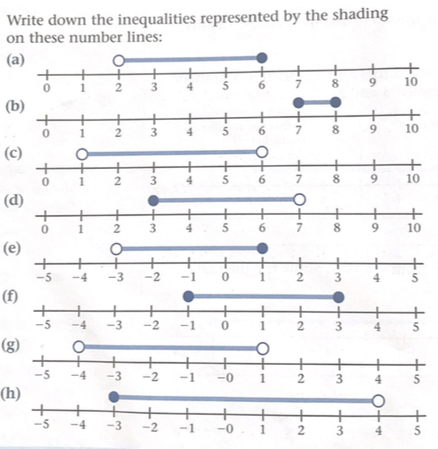Tuesday 21st April 2020

Today our focus in on inequalities and intervals.

Key vocabulary: inequality, less than, less than or equal to, greater than, greater than or equal to, interval, value

Carefully watch the lesson on MyMaths.

Then complete the online homework.

Monday 20th April 2020

Good morning everybody.

This week we are going to start handling data.

Key vocabulary: qualitative. quantitative, discrete, continuous, primary, secondary, population, census, sample.

It is important to understand that there are different types of data.

When we understand the type of data that we are we are looking at, we can then decide how we can collect, sort and present it.

Watch the MyMaths lesson.

As you watch the lesson, make notes on the different types of data in your home learning book.

Friday 17th April 2020

Good morning everybody.

Here are the answers to yesterday’s perimeter and area work.

Q1. a) 76cm
b) 72cm

Q2. a) 68 cm
b) 14 rolls are needed.

Q3. a) 40 cm2
b) 1045 cm2
c) 4340 cm2
d) 5.55 km2

Q4.   31.74 m2

Q5.   a) 54 cm2
b) 7.5 cm2
c) 87.5 cm2
d) 5.12 m2

Q6. Area of small triangle = 112.5 cm2
Area of larger triangle = 262.5 cm2
Total area = 750 cm2

Q7. 111 mm

Q8. 3937.5 mm2

Q9. a) 9.6 m
b) 9 rolls should be ordered.

Q10. 28m

Q11. Height of the triangle = 6cm

Today is our last day on area.

I would like you to start by revising area by working through the following revision page on MyMaths.

When you have finished, complete the online worksheet which I have set for you.

Well done everybody. I hope you all have a good weekend.

Thursday 16th April 2020

Today you are going to use all the skills you have learnt recently to calculate the area and perimeter of a range of shapes and solve problems.

To revise these first go to P146 of your GCSE textbook. Look at the recap section at the top of the page. Here you will find the formulae for a rectangle, parallelogram, triangle and trapezium.

Then turn to pages 74 and 75 in your GCSE AQA mathematic workbook. (The white A4 book).

Work through questions 1-11.  Read the answer tips in the bubble next to the question carefully. These are very helpful and may give you a formula or just a helpful tip.

Remember that you may need to find some missing lengths when working out the perimeter of shapes.

On Friday, I will give you the answers so that you can check your work.

Good luck.

Wednesday 15th April 2020

Today we are going to move onto finding the surface area of a range of 3D shapes.

The surface area is the total area of all the faces of a solid shape.

Watch the MyMaths lesson carefully.

You will need to think carefully about each of the faces that make up the shape.
What shape is it?
What is the formula for the area of that shape? (You jotted these down in your Home Learning book last week).

Tuesday 14th April 2020

Good morning everybody.

Today we are going to recap on finding the area of a circle.

Key vocabulary: area, pi, radius, diameter

Watch the MyMaths lesson carefully. Then have a go at the online homework.

If you do not have a scientific calculator at home, use 3.14 for pi.

Tip: Make sure you think carefully about the given measurements when calculating the area of a circle. If you have been given the diameter, don’t forget to halve the measurement because you need the radius measurement.

Thursday 9th April 2020

LO: To solve reasoning and problem solving questions linked to area

Good morning everybody, today you are going to use all the formulae that you have learnt this week to solve problems.

Open your GCSE textbooks to p146.

Read the recap section carefully. It goes through all the formulae you wil be using today.

The How To section is useful. The 4-step process will help you work through each question. Don’t forget to include the units of measurement at the end.

Read the  worked example carefully about the farmer and his two fields.

Look carefully at the second field. It is made up of 4 triangles and a trapezium. You will need to find the area of each and add it all together at the end.

What this means is that you have assumed that the given measurements are correct.  You have used these given measurements to calculate the answer. If they are not correct, the farmer may be wrong.

Now have a go at P147, Exercise 7.2A Q 1,2,3 and 4 in your home learning book.

I won’t be setting more GCSE work until Tuesday 14th April. Have a relaxing bank holiday weekend everybody.
Well done for al your hard work.

Wednesday 8th April 2020

LO: To know and apply formulae to calculate the areas of triangles, parallelograms and trapezia.

Let’s start by revising what we know:

Area of a rectangle = length x width.
Area of a parallelogram = base x perpendicular height.
Area of a triangle = ½ base x perpendicular height.
Area of a trapezium = ½ sum of the parallel lines x perpendicular height

Open your GCSE textbook to P144.

Make sure that you write down each of the steps as you work. Avoid trying to keep too much information in your head. We have discussed this!

Also, make sure that you select the perpendicular height when this measurement is needed.

Sometimes a question will give you the area. Substitute the known values into the equation and then rearrange.

Now have a go at P145, Exercise 7.2S.

Check your answers at the back of the book when you have finished.

If you are wrong, try to unpick what you have done.
Have you selected and written the correct formula?
Have you substituted the correct values into the formula? Be particularly careful with the perpendicular height.
Don’t forget the unit of measurement at the end.

Tuesday 7th April 2020

Let’s start the morning recapping what we know about area.

Area is the amount of space inside a 2D shape.
In the metric system, area is measured in mm2, cm2, m2, km2.

Area of a rectangle = length x width.

Area of a parallelogram = base x perpendicular height.

Area of a triangle = ½ base x perpendicular height.

I hope you have made a note of each of these formulae in your Home Learning book as you will be using them over the next few days.

Today I would like you to learn how to calculate the area of a trapezium.

Watch the lesson carefully on MyMaths.

Monday 6th April 2020

Good morning everybody.

I would like to start the week by saying a particular well done to three children who have put in amazing effort with their GCSE work this week.  I have left feedback for you on MyMaths and will be adding extra stars this morning to J2stars. Keep it up!

This week we will be focusing on area.

We will be recapping on some of the prior learning, for example, finding areas of rectangles, triangles and parallelograms.

We will move on to finding areas of trapezia and then using our skills to solve reasoning and fluency problems with all the shapes.

Key vocabulary this week:

Area, mm2, cm2, m2, km2, length, width, base, perpendicular height, parallel.

Rectangle, triangle, parallelogram, trapezium.

Today we will recap on areas of triangles and parallelograms.

Watch the lesson on finding the area of a triangle carefully.

Start a page in your home learning book for noting down formulae which you will be able to refer to later in the week.

Then do the online homework.

Then watch the lesson on finding the area of a parallelogram.

Then do the online homework.

Friday 3rd April 2020

Well done to all the pupils who completed the MyMaths work on vectors.
Really impressive results!

Today I would like you to watch the lesson below carefully.

This will give you more practice using column vectors to translate shapes in four quadrants.

Use the squares in your home learning maths book to translate the shapes on slide 5.

Then have a go at the online homework.

Thursday 2nd April 2020

Good morning everybody.

Let’s recap on what we learnt yesterday about vectors.

We learnt that a vector is a way to represent a movement between two places.

Vectors can be represented in different ways:

• On a diagram
• Using letters
• As a column vector

In a column vector:
the top number is the movement right or left (x)
the bottom number is the movement up or down (y)

You can and subtract vectors.

Now turn to page 400 in your GCSE textbook.

Look at the two examples.
Read them carefully and look at the working out at the side.

Now have a go at Exercise 19.4S Q1-5 in your home learning book.

Wednesday 1st April 2020

LO: To understand and use vectors.
Key vocabulary: vector, column vector, translation, direction,

As you watch the lesson, make notes in your home learning book. For example, the number at the top of the column vector shows horizontal movement and the number at the bottom of the vector shows vertical movement.

If the top number is positive, the move is to the right,
If the top number is negative, the move is to the left.

If the bottom number is positive, the move is up.
If the bottom number is negative, the move is down.

When you have done this, complete the homework.

Tuesday 31st March 2020

Good morning children.

Today is our last day on Pythagoras’ Theorem.

Today you will be applying the theorem to calculate missing sides and solve problems.

Please go to P97 of your CGP GCSE AQA mathematics workbook and complete questions 1 to 5.

Before you start, you may want to have another go on the MyMaths activity to refresh your memory.

See if you can get 1000 points and reach the top!

Monday 30th March 2020

LO: To use Pythagoras’ Theorem to reason and problem solve

Let’s recap on what we learnt last week.

In a right angled triangle the square of the length of the hypotenuse is equal to the sum of the squares of the other two sides.

To find the length of the hypotenuse use c2 = a2 + b2. (Where c = the hypotenuse)

To find the length of a shorter side use a2 = c2 - b2 or b2 = c2 - a2 (where c = the hypotenuse)

Today you will be problem solving involving the sides of a right angled triangle.

Follow these steps to solve the problem successfully.

1.Sketch a diagram and decide which side is the hypotenuse.
2. Use Pythagoras to find the side you need.
3. Round your answer to an appropriate degree of accuracy and don’t forget to include the units.

Open your GCSE textbooks to page 390.

Look carefully at the three examples.
Pythagoras can be used to find diagonals of rectangles, how far ladders reach when propped up against a wall and distances between points on a coordinate grid. How clever is that?

Follow the steps (1,2,3,) and work through each example in your book.

Now complete Ex 19.1A p391 Q 1-8 in your Home Learning book.

Don’t forget to tell your teacher how you are finding home learning in GCSE when you fill in your home contact form. She will pass the message onto me.

Friday 27th March 2020

Good morning everybody.

Well done to the children who completed the MyMaths homework. I have added points on your j2Stars page.

We are going to continue the Pythagoras theme today and first of all I would like you to have a go at the activity below.

I hope you enjoyed it!

Now turn to page 86 in your GCSE maths foundation homework book.

Think carefully about Q3.  You have been given the hypotenuse.

How will you use it and the other measurement to find the missing side of the rectangle?

Read the question again to make sure you have answered it correctly and found the perimeter and area of each shape.

When you complete your Home Learning Contact Form, tell your teacher how your GCSE work is going. They will tell me and I can provide some extra questions on any areas of difficulty.

Thursday 26th March 2020

Yesterday we learnt about Pythagoras’ Theorem.
In a right angled triangle the square of the length of the hypotenuse is equal to the sum of the squares of the lengths of the other two sides.
So: a+ b2 = c2

Look at the examples on P388 Exercise 19.1.

It is useful to sketch the triangle into your books and label the sides.

Don’t forget that when you have squared a and b to find c,  that you will need to find the square root of c.

So: if a = 5, b = 12, c = ?

C2  = 52 + 122

C2 = 25 + 144 = 169

C = √169  = 13      Remember: √ means square root.

Now have a go at exercise 19.1S p389 of your GCSE book in your home learning book.

Wednesday 25th March 2020

Good morning everybody!

Today we are goint to learn one of my favourites: Pythagoras' Theorem.

Pythagoras was a famous Greek mathematician and philosopher. (c.570-c.495 BC)

What does c mean? It's short for circa, from the Latin meaning around. We don't know his dates exactly.

Key vocabulary for today:

Hypotenuse: the longest side of a right angled triangle.

Log into MyMaths and you will be able to do the lesson and complete the online homework.

I have left it on for two days to allow you to complete it. I will then leave feedback for each of you.

Enjoy!

Tuesday 24th March 2020

Well done to the children who had a go at the solving equations 3 on Mymaths! Impressive work!

Today I want you to work on constructing an equation using the given information.

We looked at this in GCSE on 16.3.20.

Look at this example:

Mary thinks of a number.

She adds 8 and then divides by 3. Her answer is the number she first thought of.

Find Mary's number.

How could we construct this equation?

Let the number = n

n + 8 = n

3

Remember to balance each side.

1. Multiply both sides by 3

so we get n + 8 = 3n

2. Subtract n from both sides

so we get 8 = 2n

3. Divide both sides by 2

so 4 = n

Lucy's number is 4

Now have a go at P209 Exercise 10.2A in your home learning books.

Monday 23rd March 2020

Today I would like you to carry on with the work we started last week in GCSE.

LO: To solve linear equations with unknowns on both sides.

Last week we used the balancing method to solve equations. Remember that whatever you do to one side, you must do to the other side.

As a recap, watch the lesson by following the link below:

Have another look at the examples on P206 of your GCSE textbook if you need to.